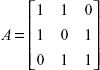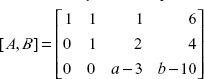## Match the Following

#### Chapter 1 Matrices and Linear Systems of Equations

 1. A is an orthogonal matrix a.1 2. A is an invertible matrix b.3 3. P(A) wherec. AAT where A is a square matrix 4. P(A) whered. AB = BA = I for some B 5. Symmetric matrix system of equations AX = B wheree. AAT = ATA = I 6. a ≠ 3, b any number f. No solution 7. a = 3, b ≠ 10 g. Finitely many solutions 8. a = 3, b = 10 h. Unique solution i. Infinite ...

Get Mathematical Methods now with the O’Reilly learning platform.

O’Reilly members experience live online training, plus books, videos, and digital content from nearly 200 publishers.